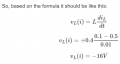# Why the voltage is positive while the current is decreasing

#### skyline977

Joined Jan 14, 2021
29
Hi all,

The question is:The solution is:The formula used is:Why did he change the formula by putting an additional negative sign in front of the formula?
Why does the voltage increase as the current decrease?

The question and answer are from: FE Exam Review: Electrical and Computer Engineering 1st Edition by Myron E. Sveum Publisher : Professional Publications, Inc. ISBN-10 : 1591260698 ISBN-13 : 978-1591260691

The formula is taken from: FE Reference Handbook 10.0.1 By NCEES ISBN 978-1-947801-11-0

My attempt to solve the question is:Last edited:

#### ericgibbs

Joined Jan 29, 2010
17,458
hi,

E

•skyline977

#### skyline977

Joined Jan 14, 2021
29
hi,

E
Done.

•Deleted member 115935

#### skyline977

Joined Jan 14, 2021
29

#### Wolframore

Joined Jan 21, 2019
2,590
You are correct it’s -16V across the inductor in relation to ground, but +16 at the negative terminal of the inductor, so if you draw a diagram with an inductor that’s in series with the source it would add voltage (notable when you place a load between inductor and ground). While this appears to be a negative voltage intuitively, it’s actually positive. I agree inductors can get confusing but very important. Just for fun figure out what happens if you reduced the time to 1uS.

•skyline977

#### crutschow

Joined Mar 14, 2008
32,090
An inductor always resists any change in current, or tries to keep the current moving in the same direction so from that, you can determine the voltage at the terminals for any conditions.aw89p[89+fg'

If it helps, inductance is the analog of inertia in the mechanical world.

#### MrAl

Joined Jun 17, 2014
10,110
Hi all,

The question is:The solution is:The formula used is:Why did he change the formula by putting an additional negative sign in front of the formula?
Why does the voltage increase as the current decrease?

The question and answer are from: FE Exam Review: Electrical and Computer Engineering 1st Edition by Myron E. Sveum Publisher : Professional Publications, Inc. ISBN-10 : 1591260698 ISBN-13 : 978-1591260691

The formula is taken from: FE Reference Handbook 10.0.1 By NCEES ISBN 978-1-947801-11-0

My attempt to solve the question is:
View attachment 229442
Hello there,

It is interesting that in a pure electronic/electrical book you would normally see v=L*di/dt and that is evident in the various literature. In a pure physics book you may see v=-L*di/dt. I think the better way to write this however would be v=L*(-di/dt) because it would indicate a change in the direction of the current not just the whole thing being made negative.
I say this because in most of electrical engineering and control theory we see v=L*di/dt being used and that is because we assume conventional current is being observed.
Now obviously the voltage measured must be of the same polarity no matter if we use conventional or electron current flow, so if we use electron current flow we would have to make the current negative and this flips the sign so that the voltage comes out the same for both cases.
That seems to be a logical explanation and i will review some of my physics books later today we just got swamped by 2 feet of snow i have to take care of first.

•skyline977

#### skyline977

Joined Jan 14, 2021
29
An inductor always resists any change in current, or tries to keep the current moving in the same direction so from that, you can determine the voltage at the terminals for any conditions.aw89p[89+fg'

If it helps, inductance is the analog of inertia in the mechanical world.
Hi, I didn't understand what you meant by ".aw89p[89+fg'" in your reply or is it by mistake? I want to know everything you write.
Thanks